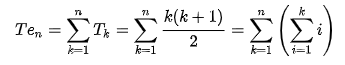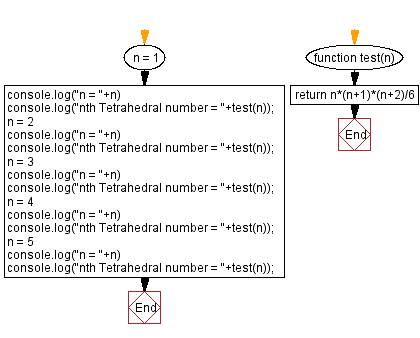# JavaScript: Nth Tetrahedral Number

## JavaScript Math: Exercise-78 with Solution

Write a JavaScript program that takes an integer n and returns the nth Tetrahedral number.

A tetrahedral number, or triangular pyramidal number, is a figurate number that represents a pyramid with a triangular base and three sides, called a tetrahedron. The formula for the nth tetrahedral number is represented by the 3rd rising factorial of n divided by the factorial of 3:The tetrahedral numbers are:
1, 4, 10, 20, 35, 56, 84, 120, 165, 220, ...

Test Data:
(1) -> 1
(2) -> 4
(3) -> 10
(4) -> 20
(5) -> 35

Sample Solution:

HTML Code:

``````<!DOCTYPE html>
<html>
<meta charset="utf-8">
<title>JavaScript program to Nth Tetrahedral Number</title>
<body>

</body>
</html>
```
```

JavaScript Code:

``````function test(n) {
return n*(n+1)*(n+2)/6
}
n = 1
console.log("n = "+n)
console.log("nth Tetrahedral number = "+test(n));
n = 2
console.log("n = "+n)
console.log("nth Tetrahedral number = "+test(n));
n = 3
console.log("n = "+n)
console.log("nth Tetrahedral number = "+test(n));
n = 4
console.log("n = "+n)
console.log("nth Tetrahedral number = "+test(n));
n = 5
console.log("n = "+n)
console.log("nth Tetrahedral number = "+test(n));
```
```

Sample Output:

```n = 1
nth Tetrahedral number = 1
n = 2
nth Tetrahedral number = 4
n = 3
nth Tetrahedral number = 10
n = 4
nth Tetrahedral number = 20
n = 5
nth Tetrahedral number = 35
```

Flowchart:Live Demo:

See the Pen javascript-math-exercise-78 by w3resource (@w3resource) on CodePen.

Improve this sample solution and post your code through Disqus

What is the difficulty level of this exercise?

Test your Programming skills with w3resource's quiz.

﻿